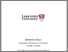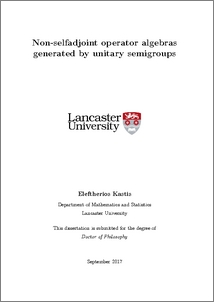# Non-selfadjoint operator algebras generated by unitary semigroups

Kastis, Eleftherios Michail (2017) Non-selfadjoint operator algebras generated by unitary semigroups. PhD thesis, UNSPECIFIED.Preview
PDF (2017KastisPhD)
2017KastisPhD.pdf - Published Version

## Abstract

The parabolic algebra was introduced by Katavolos and Power, in 1997, as the weak∗-closed operator algebra acting on L2(R) that is generated by the translation and multiplication semigroups. In particular, they proved that this algebra is reflexive, in the sense of Halmos, and is equal to the Fourier binest algebra, that is, to the algebra of operators that leave invariant the subspaces in the Volterra nest and its analytic counterpart.   We prove that a similar result holds for the corresponding algebras acting on Lp(R), where 1 < p < ∞. It is also shown that the reflexive closures of the Fourier binests on Lp(R) are all order isomorphic for 1 < p < ∞.   The weakly closed operator algebra on L2(R) generated by the one-parameter semigroups for translation, dilation and multiplication by eiλx, λ ≥ 0, is shown to be a reflexive operator algebra with invariant subspace lattice equal to a binest. This triple semigroup algebra, Aph, is antisymmetric, it has a nonzero proper weakly closed ideal generated by the finite-rank operators, and its unitary automorphism group is R. Furthermore, the 8 choices of semigroup triples provide 2 unitary equivalence classes of operator algebras, with Aph and (Aph)∗ being chiral representatives.   In chapter 4, we consider analogous operator norm closed semigroup algebras. Namely, we identify the norm closed parabolic algebra Ap with a semicrossed product for the action on analytic almost periodic functions by the semigroup of one-sided translations and we determine its isometric isomorphism group. Moreover, it is shown that the norm closed triple semigroup algebra AphG+ is the triple semi-crossed product Ap ×v G+, where v denotes the action of one-sided dilations. The structure of isometric automorphisms of AphG+ is determined and AphG+ is shown to be chiral with respect to isometric isomorphisms.     Finally, we consider further results and state open questions. Namely, we show that the quasicompact algebra QAp of the parabolic algebra is strictly larger than the algebra CI + K(H), and give a new proof of reflexivity of certain operator algebras,generated by the image of the left regular representation of the Heisenberg semigroup H+.

Item Type:
Thesis (PhD)
Subjects:
Departments:
ID Code:
88135
Deposited By:
Deposited On:
06 Oct 2017 20:18
Refereed?:
No
Published?:
Published